# Simply-supported Square PlateThis test is used to study the convergence and the absence of any locking in the case of very thin shells (th / L = 0.5e-4).
You will use 2D meshes.Reference:

TIMOSHENKO S.P., WOINOWSKY-KRIGER S., Theory of Plates and Shells, 1959.

##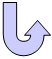Specifications

### Geometry Specifications

 Length: L = 200 mm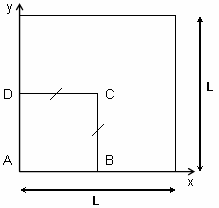Thickness: th = 0.01 mm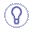For symmetry reasons, only a quarter of the plate is modeled (ABCD).

### Analysis Specifications

 Young Modulus (material): E = 78000 MPa Poisson's Ratio (material): ν= 0.3 Mesh Specifications: Imposed numbers of nodes (see the tables of results)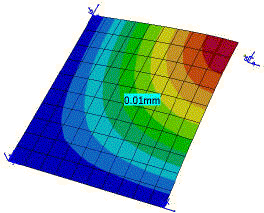Restraints (User-defined): Point A (position fixed): Tx=Ty=0 Edge AB (simply supported edge): Tz=Ry=0 Edge DA (simply supported edge): Tz=Rx=0 Edge BC (symmetry plane edge): Tx=Ry=Rz=0 Edge CD (symmetry plane edge): Ty=Rx=Rz=0 Loads: Case 1 (Distributed force): Fz = 0.001 N at point C(equivalent to a concentrated vertical force) Case 2 (Pressure): P=0.1 Pa on the plate

##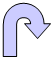Results

• The analytical solutions for the z displacement of the point C are:
Case 1: Tz=64.96 mm
Case 2: Tz=90.942 mm

• The tables below present the analysis results for cases 1 and 2.
The normalized results (computed results divided by analytical solution) are listed.

• Case 1:

 Nodes Analytical solution  [mm] Values Linear triangle (TR3) Parabolic triangle (TR6) Linear quadrangle (QD4) Parabolic quadrangle (QD8)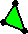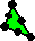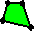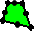Computed results  [mm] Normalized results Computed results  [mm] Normalized results Computed results  [mm] Normalized results Computed results  [mm] Normalized results 3 x 3 64.96 62.15 0.957 62.09 0.956 67.44 1.038 63.03 0.970 5 x 5 64.96 64.66 0.995 64.61 0.995 66.37 1.022 64.58 0.994 7 x 7 64.96 64.81 0.998 64.83 0.998 65.77 1.013 64.80 0.997 9 x 9 64.96 64.87 0.999 64.89 0.999 65.49 1.008 64.87 0.999 11 x 11 64.96 64.91 0.999 64.92 0.999 65.33 1.006 64.91 0.999
• Case 2:

 Nodes Analytical solution  [mm] Values Linear triangle (TR3) Parabolic triangle (TR6) Linear quadrangle (QD4) Parabolic quadrangle (QD8)Computed results  [mm] Normalized results Computed results  [mm] Normalized results Computed results  [mm] Normalized results Computed results  [mm] Normalized results 3 x 3 90.942 83.621 0.919 87.039 0.957 90.308 0.993 86.767 0.987 5 x 5 90.942 89.196 0.981 91.117 1.002 91.576 1.007 90.977 1.000 7 x 7 90.942 90.276 0.993 91.053 1.001 91.366 1.005 90.998 1.001 9 x 9 90.942 90.596 0.996 91.030 1.001 91.231 1.003 90.998 1.001 11 x 11 90.942 90.746 0.998 91.019 1.001 91.155 1.002 90.998 1.001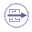To Perform the Test:

The simplysupportedplate11nodesqd8.CATAnalysis document presents a complete analysis of this case, computed with a mesh formed of 11 nodes and parabolic quadrangle elements (QD8).

To compute the case with other types of elements and other number of nodes, proceed as follow:

1. Open the CATAnalysis document.

2. In the Advanced Meshing Tools workbench, change the mesh specifications as indicated above.

3. Compute the case in the Generative Structural Analysis workbench.